# Calculation is SSAS (SQL Server Analysis Service)

The error here occurred while doing the SSAS calculations.

### Error:

Below image is the snapshot from SSAS cube where we are doing calculation and while we are dragging and dropping the measure & dimension fields we cannot see the calculations.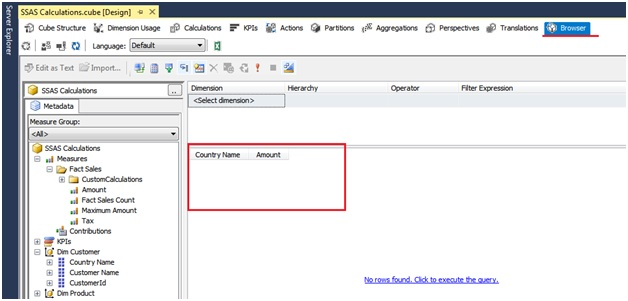There is a pre calculated script which is present when we make the cube and dimensions due to which we can see the basic calculations. But here as we see that calculated script is missing.

### Solution:

Now as we know that at the time of making cube and dimensions the calculated script is generated at that time itself. Now during MSBI offline training one candidate somehow accidentally deleted the “Calculated” script the cube will not work and therefore we encounter the error as shown above. So we have to make sure that the Default Calculate script is never deleted.

The Default Calculate script command helps to calculate aggregation of cube.

Now if the calculated script is deleted then we can create a new one once again,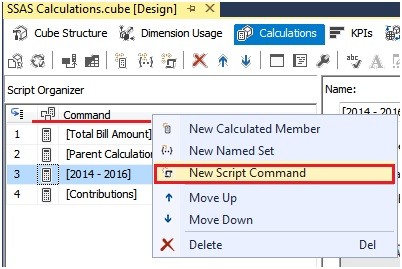Or we can do in this way, click on the icon as shown below which is available under Menu bar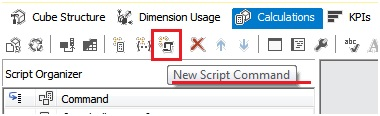Then, we simply write “Calculate”in the script window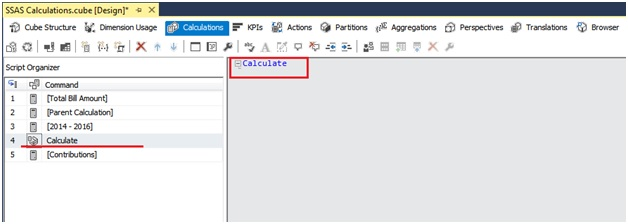And then save and deploy the solution by clicking on Build > Deploy Solution as shown below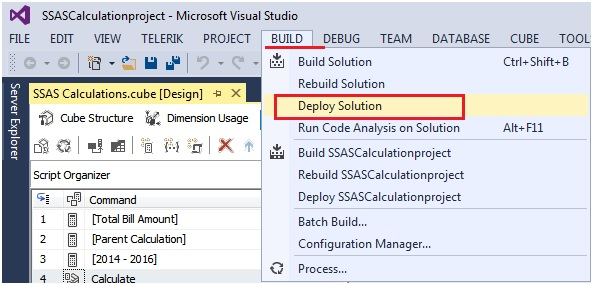After deploying go to the browser, reconnect the browser and then try to take the amount measure and any dimension and we will see the aggregated calculation.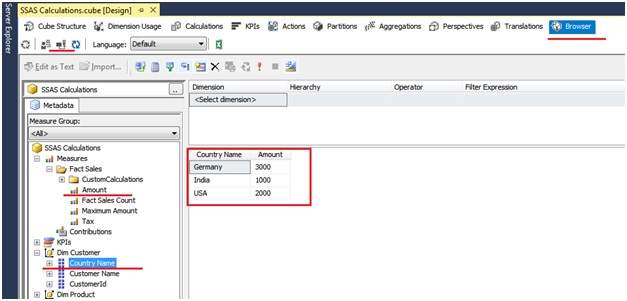This issue was practical learning example for other candidate of MSBI training whose unknowingly done mistake helped others to learn as well. Likewise there are other such mistake done and are resolved along with the training syllabus.